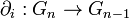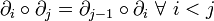# Simplicial group

(diff) ← Older revision | Latest revision (diff) | Newer revision → (diff)
This is a variation of group|Find other variations of group | Read a survey article on varying group
QUICK PHRASES: simplicial object in category of groups, group object in category of simplicial sets

## Definition

### Abstract definition

A simplicial group can be defined in the following equivalent ways:

1. Explicitly, it is a contravariant functor from the simplex category to the category of groups.
2. It is a simplicial object in the category of groups.
3. It is a group object in the category of simplicial sets.

### Explicit definition

A simplicial group$G$ is the following data:

1. For every nonnegative integer, a group$G_n$
2. For every positive integer$n$ and all$i$ with$0 \le i \le n$, a group homomorphism$\partial_i:G_n \to G_{n-1}$ called a face map
3. For every nonnegative integer$n$ and all$i$ with$0 \le i \le n$, a group homomorphism$\sigma_i:G_n \to G_{n+1}$ called a degeneracy map

(Note that there are actually multiple maps$\partial_i$ and$\sigma_i$ corresponding to different values of$n \ge i$. However, on any given$G_n$, there is only one map, so we can typically avoid specifying the$n$-value).

with the following conditions:

1.$\partial_i \circ \partial_j = \partial_{j-1} \circ \partial_i\ \forall \ i < j$ for each$n$ where either side makes sense
2.$\sigma_i \circ \sigma_j = \sigma_{j+1}\circ \sigma_i \ \forall \ i \le j$ for each$n$ where either side makes sense
3.$\partial_i \circ \sigma_j = \sigma_{j-1} \circ \partial_i \ \forall \ i < j$ for each$n$ where either side makes sense
4.$\partial_i \circ \sigma_j = \operatorname{id} \ \forall \ i = j \mbox{ or } i = j + 1$ for each$n$ where either side makes sense
5.$\partial_i \circ \sigma_j = \sigma_j \partial_{i-1} \ \forall \ i > j + 1$ for each$n$ where either side makes sense

Note that all these conditions follow from the relations originally present in the simplex category. They can be constructed systematically using the trapezoid rule.